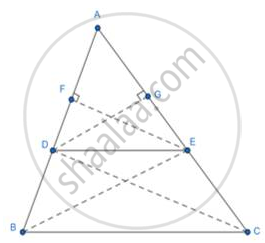# Prove That, If a Line Parallel to a Side of a Triangle Intersect the Other Sides in Two District Points, Then the Line Divides Those Sides in Proportion. - Geometry

Sum

Prove that, if a line parallel to a side of a triangle intersects the other sides in two district points, then the line divides those sides in proportion.

#### Solution

Given: A Δ ABC in which DE||BC, and intersect AB in D and AC in E.

Prove: "AD"/"BD" = "AE"/"EC"

Construction: Join BE, CD and draw EF BA and DGCA.Proof:

Area ∆ADE = 1/2 xx ("base" xx "height") =1/2("AD. EF")

Area ∆DBE = 1/2 xx("base" xx "height") =1/2("DB.EF")

⇒  ("Area"(triangle"ADE"))/("Area"(triangle"DBE"))=[((1/2"AD. EF"))/(1/2"DB. EF"]] = "AD"/"DB"

Similarly,

("Area"(triangle"ADE"))/("Area"(triangle"DBE"))=[((1/2"AE. DG"))/(1/2"EC. DG"]] = "AE"/"EC"

ΔDBE and ΔDEC are on the same base DE and b\w the same parallel DE and BC.

Area (Δ DBE) = Area (ΔDEC)

⇒ 1/("Area"(triangle"DBE")) =1/("Area"(triangle"DEC"))  .....[Taking reciprocal of both sides]

⇒ ("Area"(triangle"ADE"))/("Area"(triangle"DBE"))=("Area"(triangle"ADE"))/("Area"(triangle"DEC")  ......[Multiplying both sides by Area (Δ ADE)]

⇒ "AD"/"DB"="AE"/"EC"

Hence Proved.

Concept: Property of three parallel lines and their transversals
Is there an error in this question or solution?